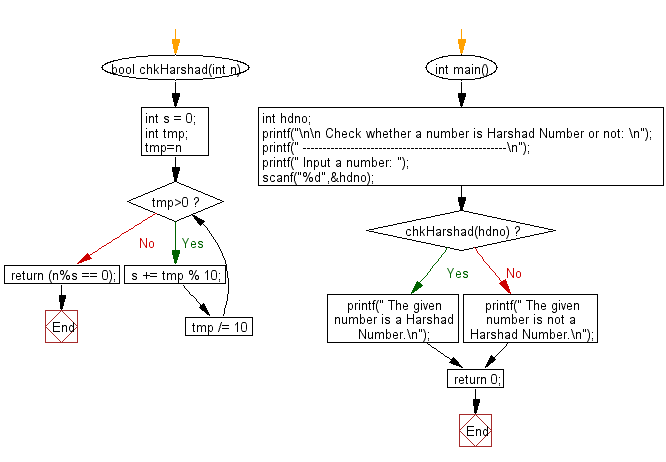﻿ C Program: Check if a number is Harshad Number or no - w3resource# C Exercises: Check if a number is Harshad Number or not

## C Numbers: Exercise-17 with Solution

Write a program in C to check if a number is Harshad Number or not.

Test Data
Input a number: 9

Sample Solution:

C Code:

``````# include <stdio.h>
# include <stdlib.h>
# include <stdbool.h>

{
int s = 0;
int tmp;
for (tmp=n; tmp>0; tmp /= 10)
s += tmp % 10;
return (n%s == 0);
}
int main()
{
int hdno;
printf("\n\n Check whether a number is Harshad Number or not: \n");
printf(" ---------------------------------------------------\n");
printf(" Input a number: ");
scanf("%d",&hdno);

printf(" The given number is a Harshad Number.\n");
else
printf(" The given number is not a Harshad Number.\n");
return 0;
}
```
```

Sample Output:

``` Input a number: 9
The given number is a Harshad Number.
```

Pictorial Presentation:Flowchart:C Programming Code Editor:

What is the difficulty level of this exercise?

Test your Programming skills with w3resource's quiz.

﻿

## C Programming: Tips of the Day

Why do C and C++ compilers allow array lengths in function signatures when they're never enforced?

It is a quirk of the syntax for passing arrays to functions.

Actually it is not possible to pass an array in C. If you write syntax that looks like it should pass the array, what actually happens is that a pointer to the first element of the array is passed instead.

Since the pointer does not include any length information, the contents of your [] in the function formal parameter list are actually ignored.

Ref : https://bit.ly/3fhlvdH